## The speed of a motor boat is 20 km/hr. For covering the distance of 15 km the boat took 1 hour more for upstream than downstream.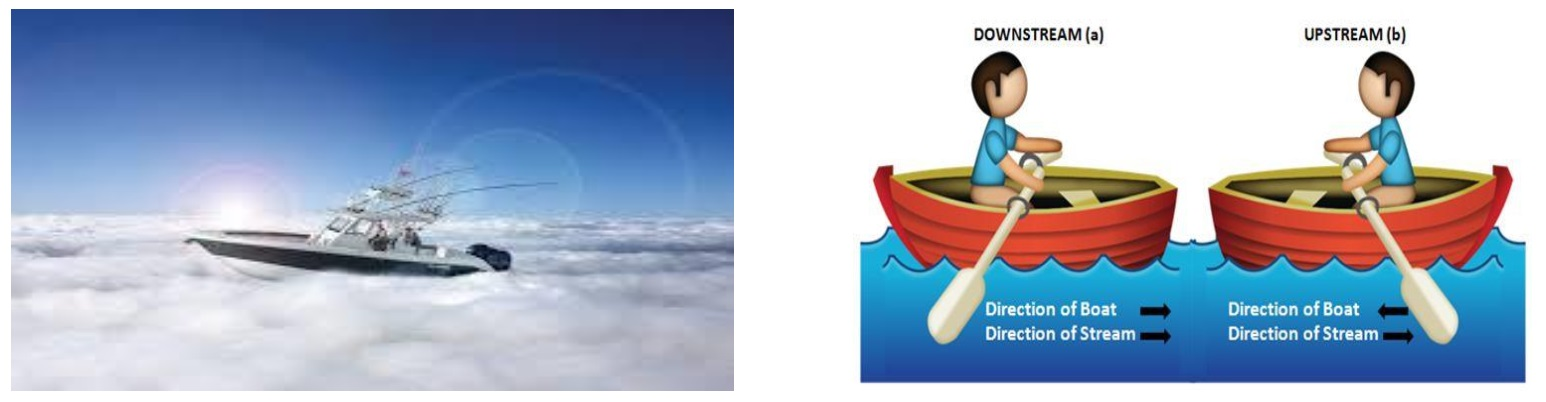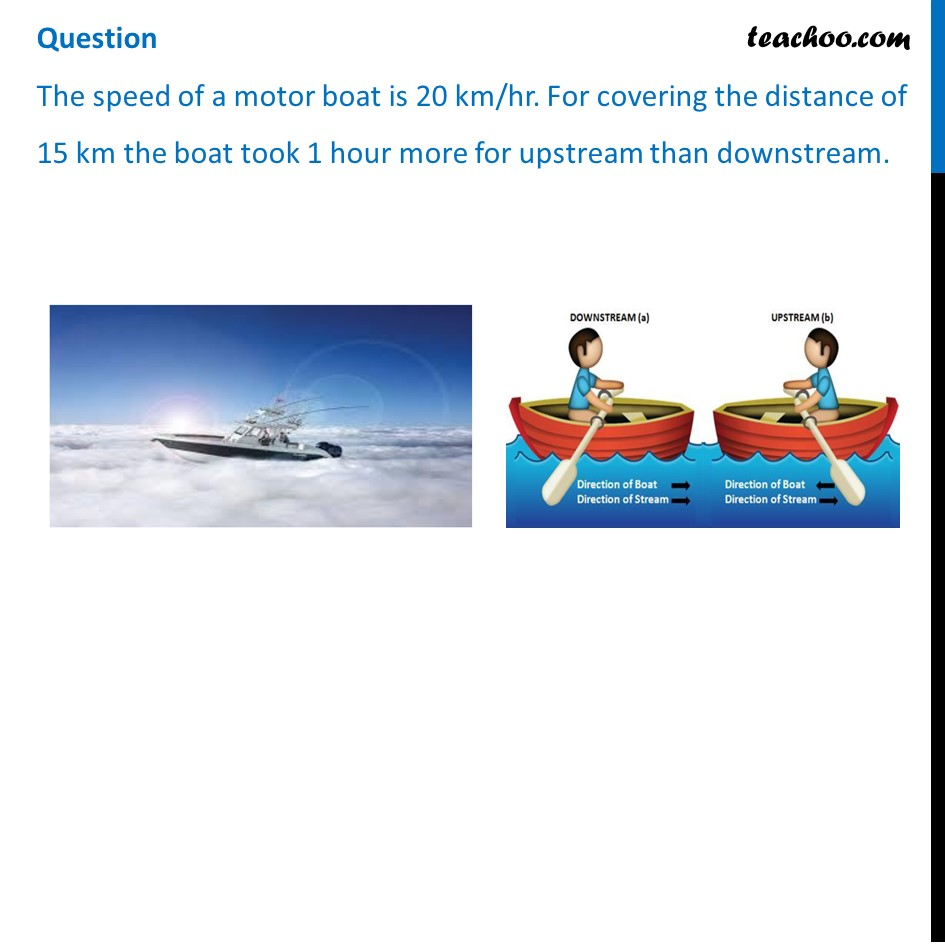## Let speed of the stream be x km/hr. then speed of the motorboat in upstream will be (a) 20 km/hr (b) (20 + x) km/hr (c) (20 – x) km/hr (d) 2 km/hr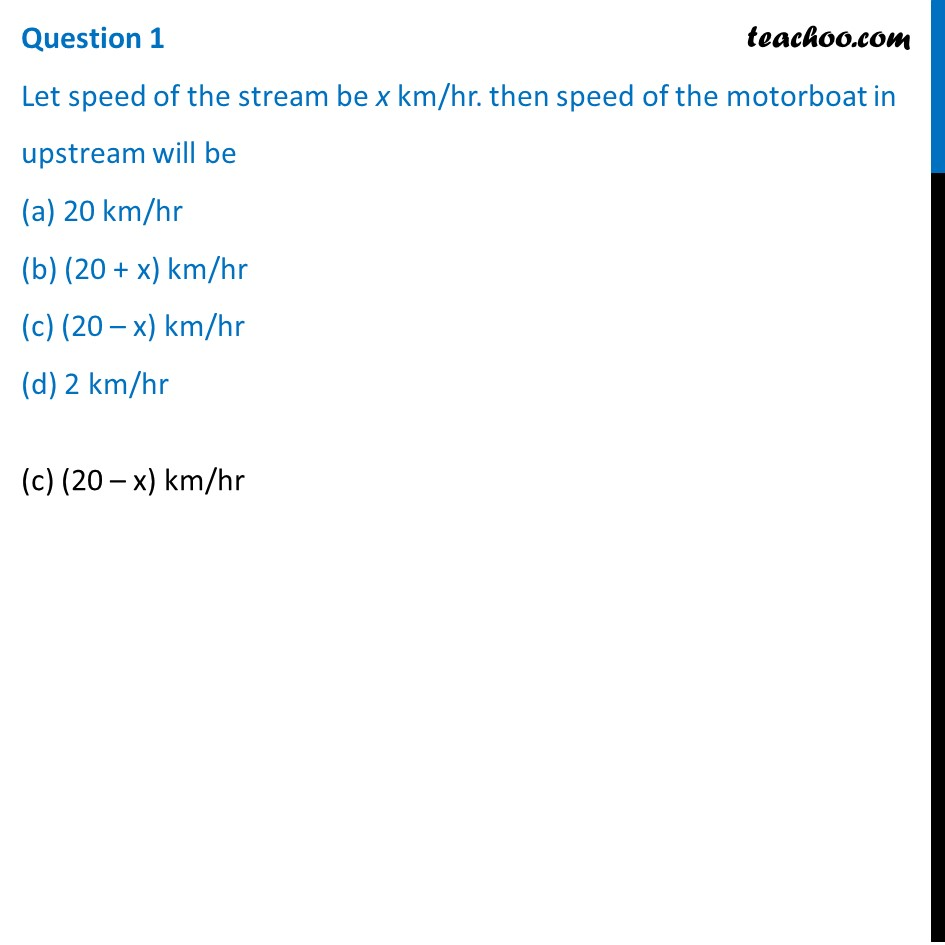## What is the relation between speed ,distance and time? (a) speed = (distance )/time (b) distance = (speed )/time (c) time = speed x distance (d) speed = distance x time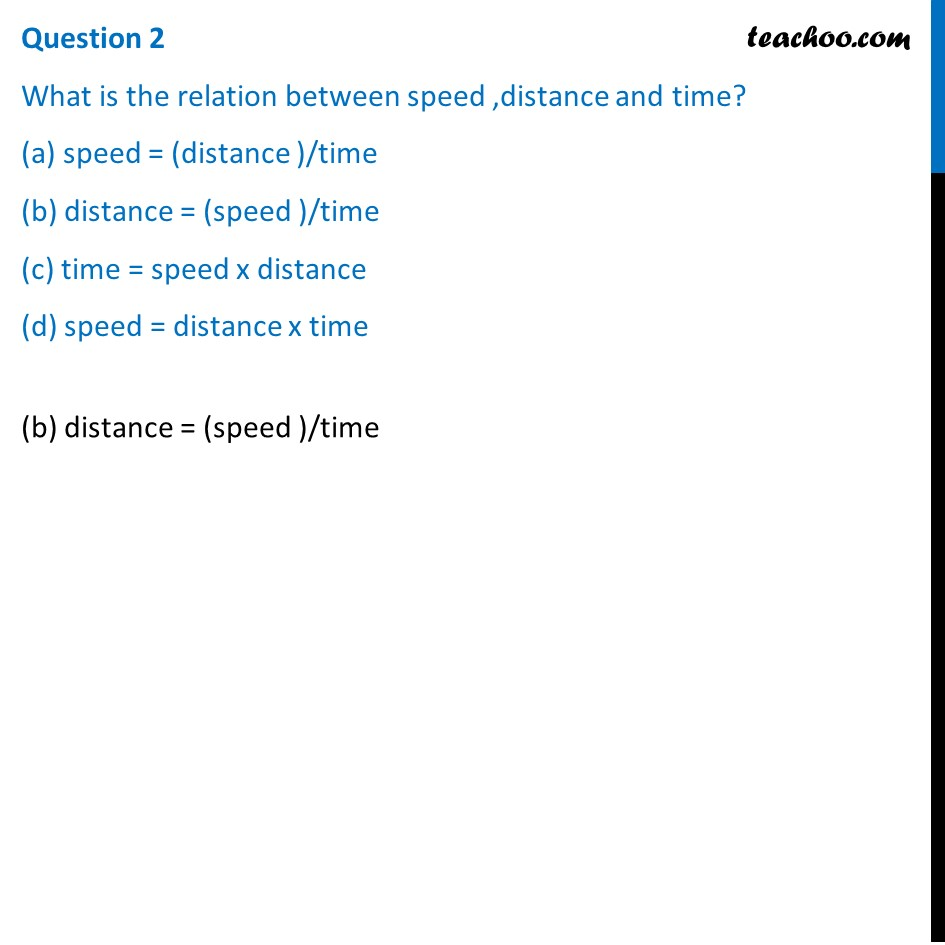## Which is the correct quadratic equation for the speed of the current ? (a) x 2 +30x-200=0 (b) x 2 +20x-400=0 (c) x 2 +30x-400=0 (d) x 2 -20x-400=0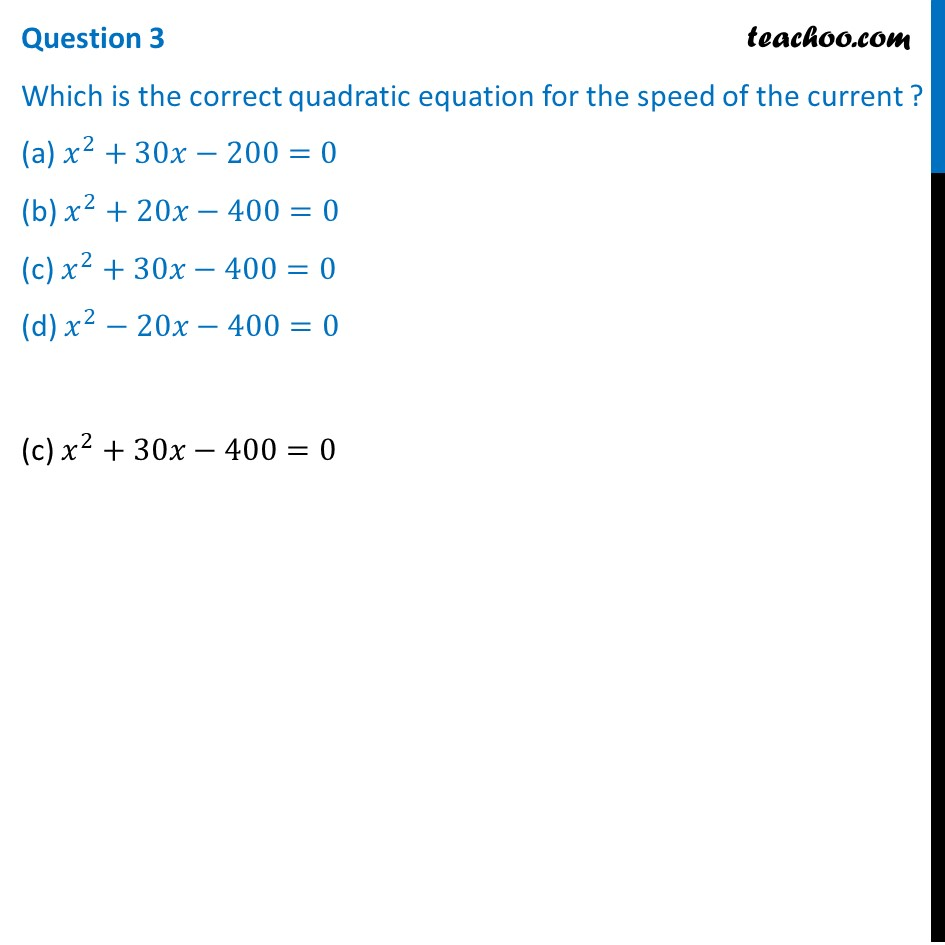## What is the speed of current ? (a) 20 km/hour (b) 10 km/hour (c) 15 km/hour (d) 25 km/hour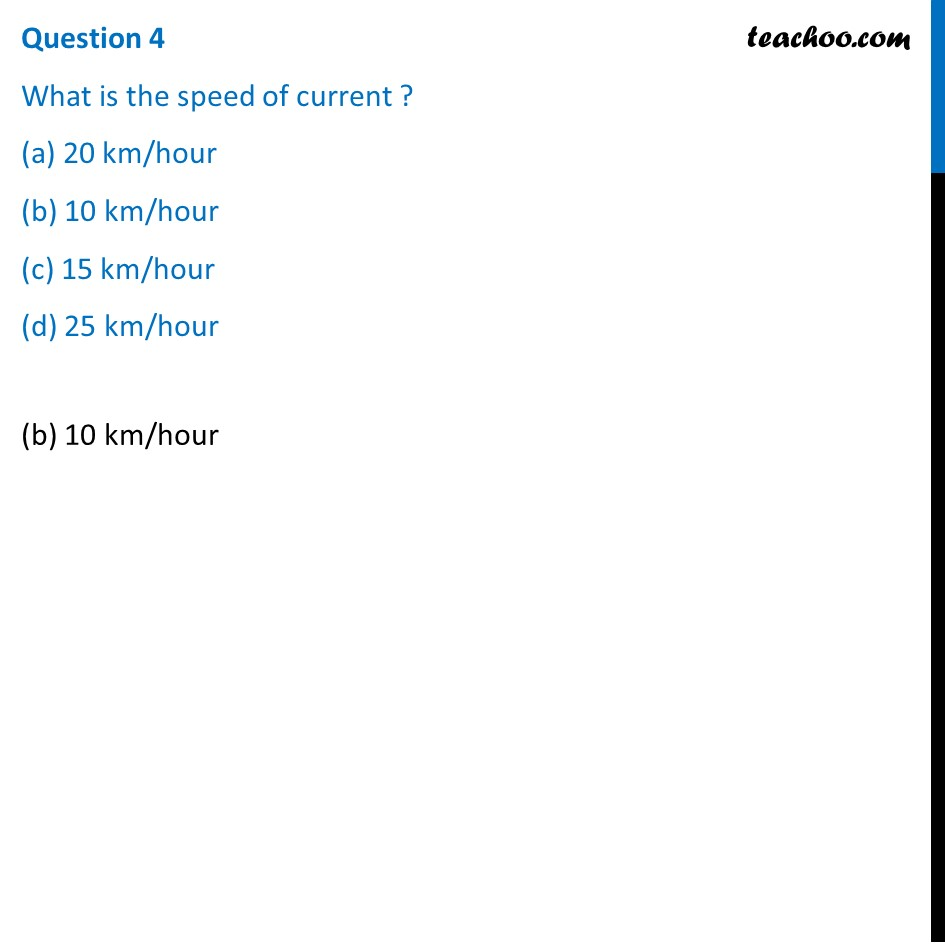## How much time boat took in downstream? (a) 90 minute (b) 15 minute (c) 30 minute (d) 45 minute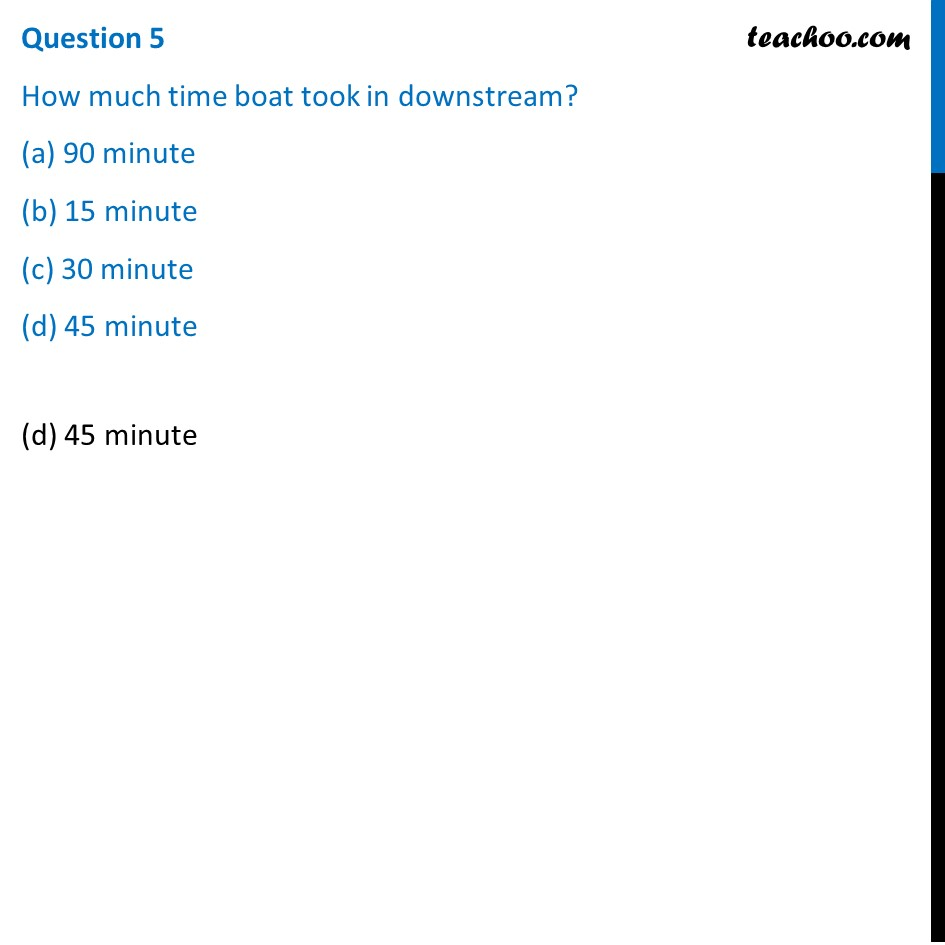1. Chapter 4 Class 10 Quadratic Equations (Term 2)
2. Serial order wise
3. Case Based Questions (MCQ)

Transcript

Question The speed of a motor boat is 20 km/hr. For covering the distance of 15 km the boat took 1 hour more for upstream than downstream.Question 1 Let speed of the stream be x km/hr. then speed of the motorboat in upstream will be (a) 20 km/hr (b) (20 + x) km/hr (c) (20 – x) km/hr (d) 2 km/hr (c) (20 – x) km/hr Question 2 What is the relation between speed ,distance and time? (a) speed = (distance )/time (b) distance = (speed )/time (c) time = speed x distance (d) speed = distance x time (b) distance = (speed )/time Question 3 Which is the correct quadratic equation for the speed of the current ? (a) 𝑥^2+30𝑥−200=0 (b) 𝑥^2+20𝑥−400=0 (c) 𝑥^2+30𝑥−400=0 (d) 𝑥^2−20𝑥−400=0(c) 𝑥^2+30𝑥−400=0 Question 4 What is the speed of current ? (a) 20 km/hour (b) 10 km/hour (c) 15 km/hour (d) 25 km/hour(b) 10 km/hour Question 5 How much time boat took in downstream? (a) 90 minute (b) 15 minute (c) 30 minute (d) 45 minute(d) 45 minute

Case Based Questions (MCQ)